# Potential at a point due to two charges

• 6Stang7

#### 6Stang7

I've been working on this problem and I cannot find out where I am making a mistake.

## Homework Statement

Two charges, each with a value of +q, are placed a distance d apart on the x-axis. Find the potential at a point P a distance z above the x-axis on the z-axis

## The Attempt at a Solution

The figure below shows the physics system: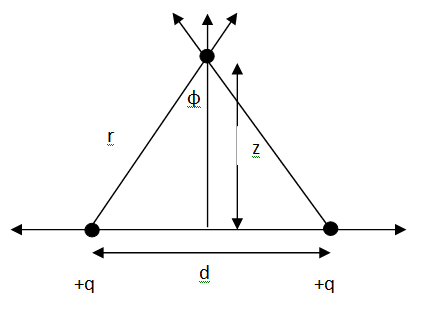The potential due to a charge is given by: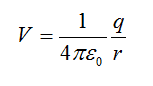We can express r as: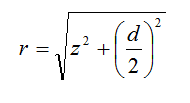We can see by symmetry that the x-components will cancel out, so the total potential will be twice of the z-component. The z-component of the potential is the cosine of the magnitude, where cosine is: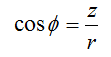This gives us: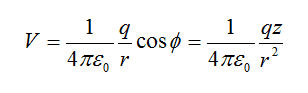Putting everything together we get: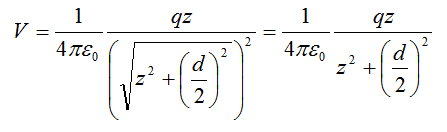Therefore, the total potential at point P is: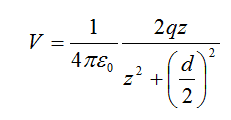Now, we know that the potential is related to the Electric Field by: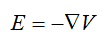This means that the Electric Field at the same point is (I realize the forget the minus sign just now):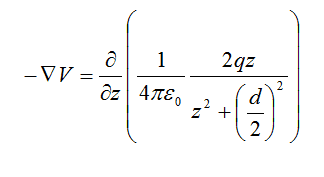Which when solved give: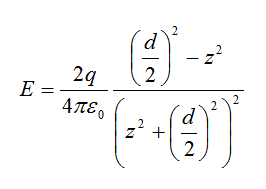Now, using the same figure, but instead of solving for the potential, we solve for the Electric Field, we find: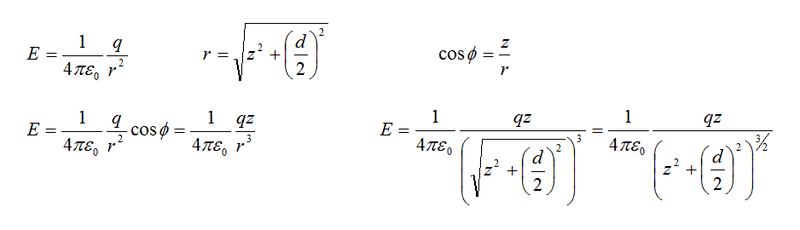The above uses the same line of thinking, and this gives a total value of the Electric Field to be: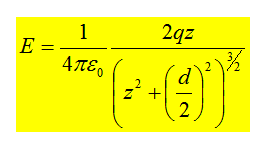The problem is that the book says that the potential I calculated above is wrong, and that the value should be: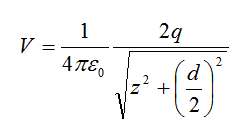When you calculate the value of the Electric Field from this, you get the same answer as the value I just calculated.

So, what did I do wrong in my calculation of the potential? The error seems to steam from the part where I calculated the z-component of one of the charges using cosine, but I can't see why that is incorrect.

I've been working on this problem and I cannot find out where I am making a mistake.

So, what did I do wrong in my calculation of the potential? The error seems to steam from the part where I calculated the z-component of one of the charges using cosine, but I can't see why that is incorrect.

Just remember that potential is not a vector, but is a scalar. You don't add scalars vectorially.

So if one of the charges was negative instead of positive, the potential would be zero?

What confuses me is that if one charge was changed to negative, then there'd be a constant potential in the x-direction, but zero in the z-direction.

So if one of the charges was negative instead of positive, the potential would be zero?

Yes, on the z axis it would be.

What confuses me is that if one charge was changed to negative, then there'd be a constant potential in the x-direction, but zero in the z-direction.
Remember, potential does not have a direction since it is a scalar and not a vector. There will be a force, or equivalently an electric field (which is force per unit charge) in the direction you say.

If potential is referenced to infinity, then the work done to bring a charge from infinity to any finite point on the z axis is clearly zero. Why, because you can chose the z axis itself as the path, and it's clear that the dot product of the electric field and the path is always zero. The x-component of electric field (and force) is orthogonal to the chosen path. The fact that electric fields are conservative tells you that any other path will yield the same value.